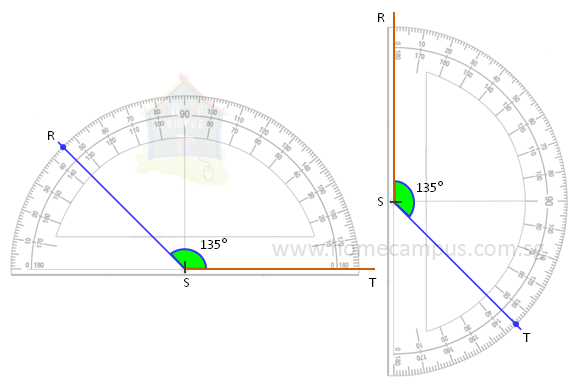## How to Drawing Angles Using Protractor?

Practice Unlimited Questions

#### 1. Draw an angle of 60°.

Step 1:
Draw a straight line. Call it AB. Mark a point on AB and call it O.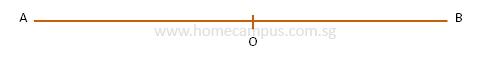Step 2:
Place the protractor's base line over AB with its centre on O.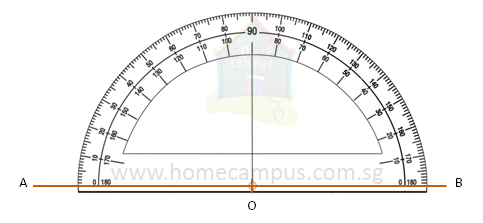Step 3:
Reading the scale from left, mark Point C so that AOC is 60°.
Join Point C to Point O and mark AOC as 60°.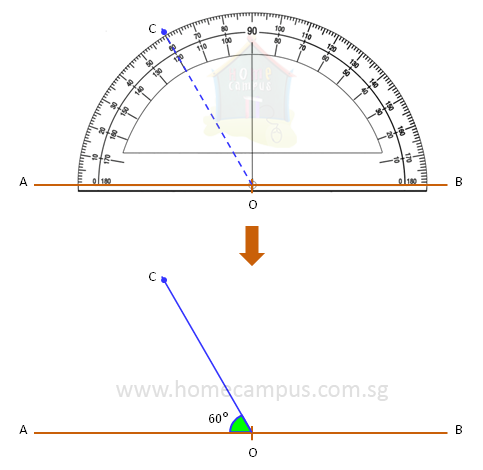Alternatively, you can read the scale from right and mark Point D so that BOD is 60°.
Join Point D to Point O and mark BOD as 60°.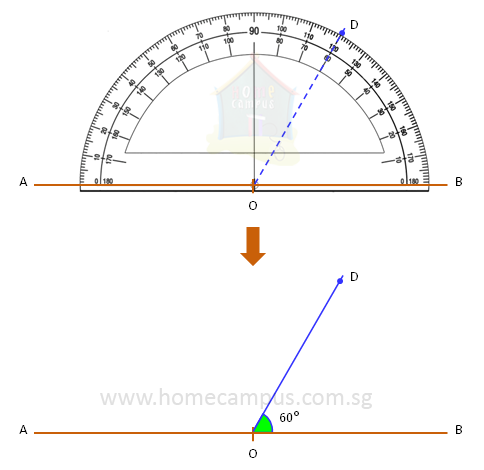#### 2. Draw ∠RST equal to 135°.

Step 1:
Draw a straight line RS.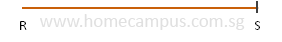Step 2:
Place the protractor's base line over RS with its centre on S.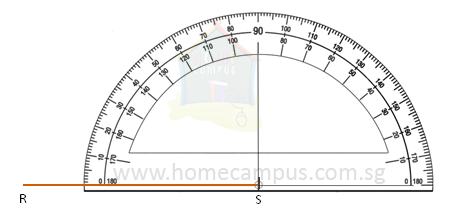∠RST tells us that the vertex of the angle is Point S.
Step 3:
Read the scale from 0° at R and mark Point T so that ∠RST is 135°.
Join Point S to Point T and mark RST as 135°.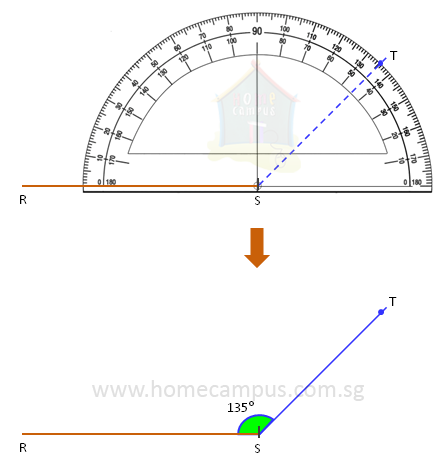Here are two more ways of drawing ∠RST equal to 135°.